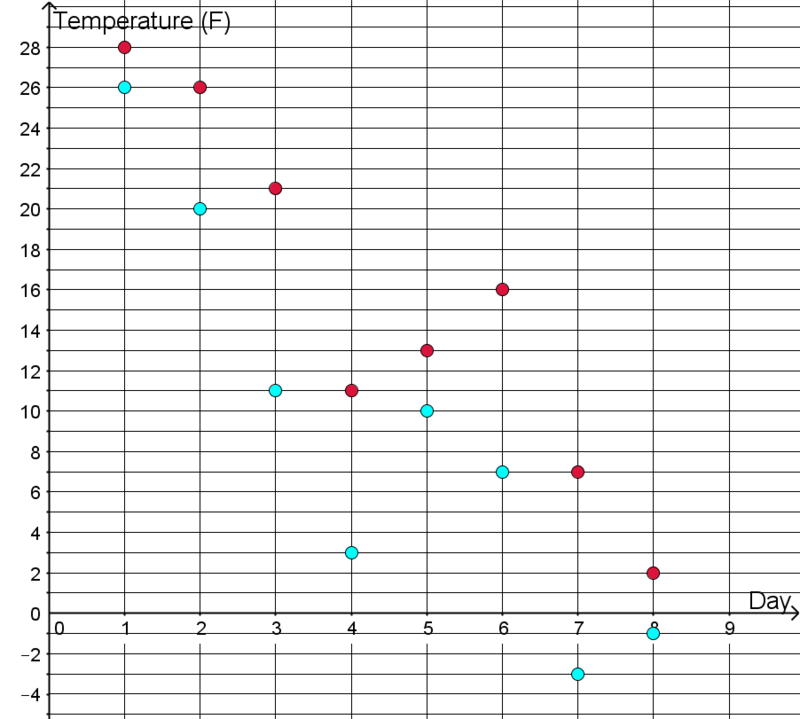##### Engage your students with effective distance learning resources. ACCESS RESOURCES>>

Alignments to Content Standards: 6.NS.C.8

The high and low temperatures, in degrees Fahrenheit, are plotted in the coordinate plane for 8 days in Nome, Alaska.1. What was the warmest high temperature?
2. What was the coldest low temperature?
3. What was the biggest same-day difference between the high and low temperature? On what day did it occur?

## IM Commentary

The purpose of this task is for students to solve a real-world problem by interpreting and comparing points in the coordinate plane. This task focuses students' attention on the y-values of the points, asking for the greatest y-value and the least y-value, as well as the greatest difference between y-values when the x-values are the same. Students should be solving similar problems that focus on the x-values, as well as problems that ask about both x- and y-values. Note that comparisons in grade 6 are limited to points that have one coordinate the same. In grade 6, students do not need to understand that the difference corresponds to a subtraction expression; they should be using the geometry of the coordinate plane to answer the questions.

Temperature is one of the best contexts to introduce ideas about signed numbers to students, because the experience of hotter and colder are nearly universal, and both Celsius and Fahrenheit temperature scales have a zero value for which negative values are possible to experience. The Fahrenheit scale has the advantage of being familiar to students in the US; the Celsius scale has the advantage that more people have real-life experience with negative values. Students should have opportunities to build up both their intuition and their ability to abstract about each scale (MP2).

## Solution

1. $28^o F$
2. $-3^o F$
3. There is a $10$ degree difference between the high and low temperature on days $3$ and $7$.